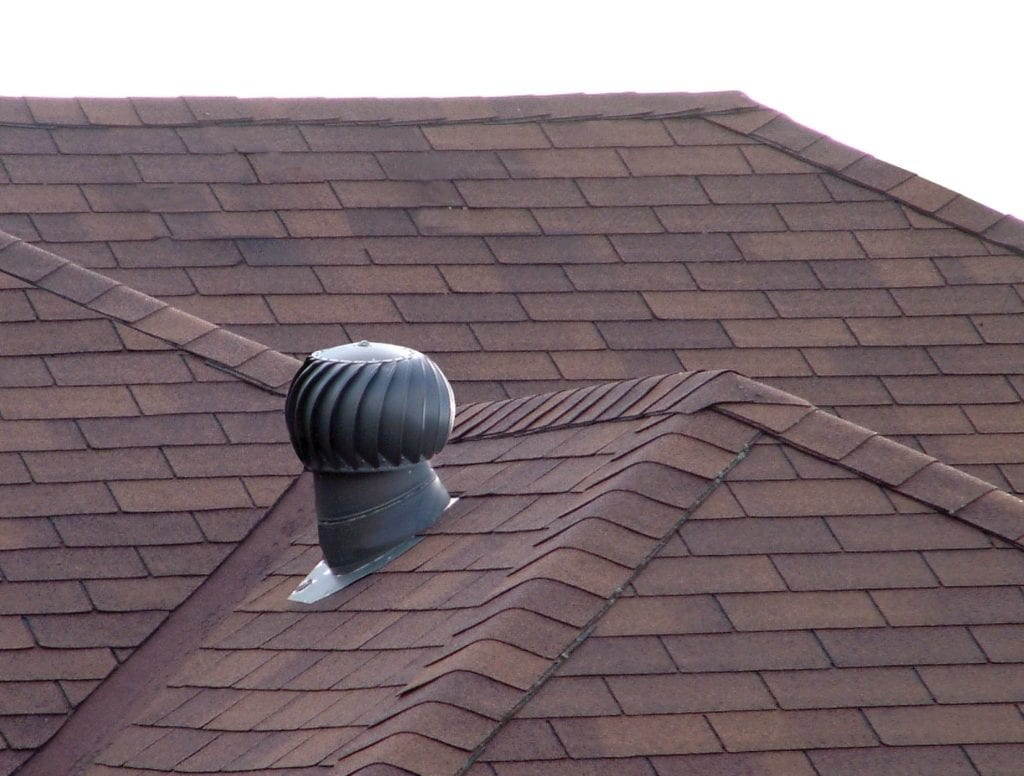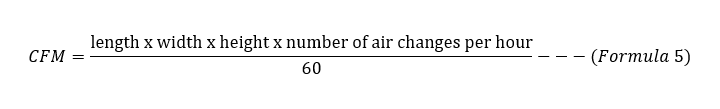# How to Size a Solar Attic Fan – CFM Calculation Explained

Generally, a quick way to size the attic venting capacity or CFM of the solar attic fan you need for your attic space is to multiply the floor area or square feet of attic space by 0.7 or 0.84, depending on the steepness of the roof.

Going by the above:

CFM for normal/flat roof = attic square footage x 0.7 — (Formula 1)

CFM for steep roof = attic square footage x 0.84 — (Formula 2)

Note that attic floor area or square footage = length x width.

In other words, if you have a 1000-square-feet attic with a 3/12 roof slope, a rough estimate of the venting capacity or size of the solar attic fan you need goes as follows:

1000 x 0.7 = 700 CFM

Conversely, if you have the same 1000-square-feet attic with a 9/12 roof slope, a rough estimate of the venting capacity of the solar attic fan you need would be:

1000 x 0.84 = 840 CFM

If the 1000-square-feet attic with a 3/12 slope had a dark roof, its estimated CFM would be:

700 x 1.15 = 805 CFM

Then, if the 1000 sq. ft. attic with 9/12 slope had a dark roof, its estimated CFM would be:

840 x 1.15 = 966 CFM

CFM is short for cubic feet per minute – it defines the volume/cubic feet of air a solar attic fan can push out in one minute. In other words, CFM defines the capacity of solar attic fans.

## Why Attics With Dark Roof Need Solar Fans With Larger Capacity?

If you have a dark roof, then you need to add a multiplier of 1.15 to any of the above formulas (Formula 1 and Formula 2) that apply to you. This holds because such roofs absorb more heat than roofs of brighter colors. And the heat they absorb leaks into the attic area, making the place even hotter.

## Why Do Attics With Steep Roof Need Attic Fans With Higher Capacity Than Those With Flat Roof?From the values above, you can tell that attics with steep roofs need solar-powered attic fans with higher CFM than flat roofs. This holds because an attic with a steep roof has more air space than a relatively flat roof.

In other words, steep-roofed attics retain more air than those with flatter roofs, and they will need stronger attic ventilation to push out the extra air.

## How to Size Solar Attic Fans for You Attic?

While the formulas or calculations mentioned in the introduction are commonplace amongst solar fans installers, there are actually some extra steps to follow to precisely determine the capacity of the attic fan you need for your attic.

### Calculate the Volume of the Attic

The first step in figuring out the size of the solar-powered attic fan you need in your attic is to determine the volume of your attic in cubic feet.

To calculate the volume of your attic, multiply the length x width x height. So, for instance, if the length of your attic space is 50 feet, while the width is 30 feet, and the height is 8 feet, the volume will be as follows:

Volume of the attic = length x width x height = 50 x 30 x 8 = 12,000 cubic feet — (Formula 3)

### Decide the Number of Air Changes Needed Every Hour

Generally, the number of air changes an attic needs per hour falls between 6 and 8. However, it all depends on how hot the attic gets. Obviously, the hotter your attic gets, the more air changes it would need to get cool.

For the 12,000 cubic feet attic above, if we decide to go with 8 air changes every hour, we can calculate the CFM using the following formula:So, for our 12,000 cubic feet attic, the CFM or attic fan size needed will be:

(12,000 x 8)/60 = 96,000/60 = 1600 CFM

#### Why People Use the Formulas We Mentioned in the Introduction

Formula 1 and Formula 2 are like an abridgment of the formula above. But to come up with that abridgment, a few assumptions were made:

• In many cases, attics have a height of 7 feet. So, Formula 1 assumes that the attic height is 7 feet.
• Formula 1 also assumes that the attic needs 6 air changes every hour.

If we expand Formula 4, we can write it as follows:So, if we assume the height is 7 feet and the number of air changes is 6, we can rewrite Formula 5 as:

CFM = (length x width x 7 x 6)/60 = length x width x 0.7 — (Formula 6)

Remember we said attic floor area = length x width? So, if we replace ‘length x width’ with attic floor area, we have:

CFM = attic square footage x 0.7 — same as Formula 1

For an attic with a steep roof, you need an additional 20% CFM. So, Formula 1 becomes:

CFM = attic square footage x 0.7 x 1.2 = attic square footage x 0.84 — same as Formula 2

## Air Intake Requirements

Attic ventilation is only possible when there is sufficient hot air extraction and cold air intake. While solar attic fans facilitate hot air extraction, air intake vents like soffit vents and gable vents bring cool air.

For proper attic ventilation, the inflow of cool air by the vent must match the extraction of hot air by the attic fan. In other words, you need to install enough soffit vents or gable vents to match your attic fan size.

How do you know you know you have enough air intake vents for your attic fan? Well, divide the CFM of the fan by 300, and you will get the minimum vent space the fan needs in sq. feet.

So, for instance, if the fan has a CFM of 1200, the minimum vent space it needs would be 1200 ÷ 300 = 4 sq. ft. If all your vents cover a 4 sq. ft. area or more, then you have sufficient vents.

While we are at it, never use ridge vents as intake vents. Ridge vents are best used as exhaust vents.

## Chart of Attic Fan Square Footage and Size Solar Attic Fan Needed

For quick reference, follow the charts below:

## How Many CFM Do I Need for a Whole House Fan?

You can calculate the number of CFM you need for a whole house fan with Formula 4 above. All you have to do is replace “volume of the attic” with “volume of the house.” So, the formula becomes:

CFM = (volume of the house x number of air changes per hour)/60

You can calculate the volume of the house the same way you calculate the volume of the attic: by multiplying the length, width, and height of the floor of your home.

For instance, if your home has a floor-length of 60 feet, a floor width of 30 feet, and a ceiling height of 8 feet, its volume would be:

volume of the house = 60 x 30 x 8 = 14,400 cubic feet

For the whole house, you will need as many as 30 air changes every hour. So, for our 14,400-cubic feet home, the CFM needed will be:

CFM = (14,400 x 30)/60 = 7200 CFM

## Final Take

To get a quick estimate of the solar attic fan size (CFM) you need for your attic, all you have to do is multiply the attic floor area by 0.7. If the roof is steep, multiply the floor area by 0.84. Then if the roof, whether steep or flat, is dark, multiply the CFM you get by 1.15.

If you want a more precise value for the attic fan size (CFM) you need for your attic, use Formula 4 above.